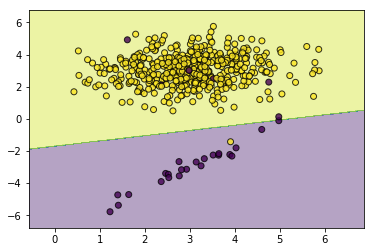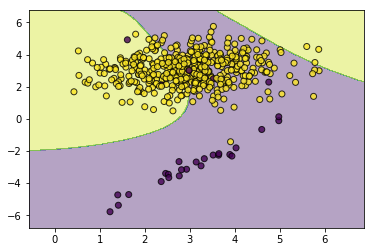### 简介¶

$$L(w,x,y)=\sum_{i=1}^Nl(w,x_i,y_i)$$

$$l(w,x_i,y_i)=(y_i-w^Tx_i)^2$$

$$l(w,x_i,y_i)=-y_ilog\phi(x_i,w)-(1-y_i)log(1-\phi(x_i,w)),这里 \phi(x,w)=\frac{1}{1+e^{-w^Tx}}$$

$$l(w,x_i,y_i)=max\{0,-y_iw^Tx_i\}$$

$$l(w,x_i,y_i)=\frac{exp(\sum_{j=1}^nw_jf_j(x_i,y_i))}{\sum_yexp(\sum_{j=1}^nw_jf_j(x_i,y))},这里f_j,j=1,2,..,n表示特征函数$$

$$l(w,x_i,y_i)=\frac{1}{2N}w^Tw+C\varepsilon_i,y_i(w^T\phi(x_i)+b)\geq 1-\varepsilon_i,\varepsilon_i\geq0$$

### 代价敏感¶

$$L(w,x,y)=\sum_{i=1}^N\alpha_il(w,x_i,y_i)$$

### 代码实现¶

（1）若采用的随机梯度下降，可以对每次更新时的梯度$dw$乘以$\alpha_i$；

（2）若采用对偶方式求解，则对其拉格朗日乘子做调整

In :
from sklearn.datasets import make_classification
import matplotlib.pyplot as plt
import numpy as np
import os
os.chdir('../')
from ml_models import utils
from ml_models.svm import SVC
%matplotlib inline

In :
X, y = make_classification(n_samples=500, n_features=2,
n_informative=2,n_redundant=0,
n_repeated=0, n_classes=2,
n_clusters_per_class=1,weights=[0.05, 0.95],
class_sep=3,flip_y=0.05, random_state=0)

In :
svc_without_sample_weight=SVC(kernel='rbf',gamma=2.0,tol=0.01)
svc_without_sample_weight.fit(X,y)
utils.plot_decision_function(X=X,y=y,clf=svc_without_sample_weight)In :
#然后我们加大少数类的权重
weights=np.where(y==0,20,1)
svc_with_sample_weight=SVC(kernel='rbf',gamma=2.0,tol=0.01)
svc_with_sample_weight.fit(X,y,sample_weight=weights)
utils.plot_decision_function(X=X,y=y,clf=svc_with_sample_weight)In [ ]: